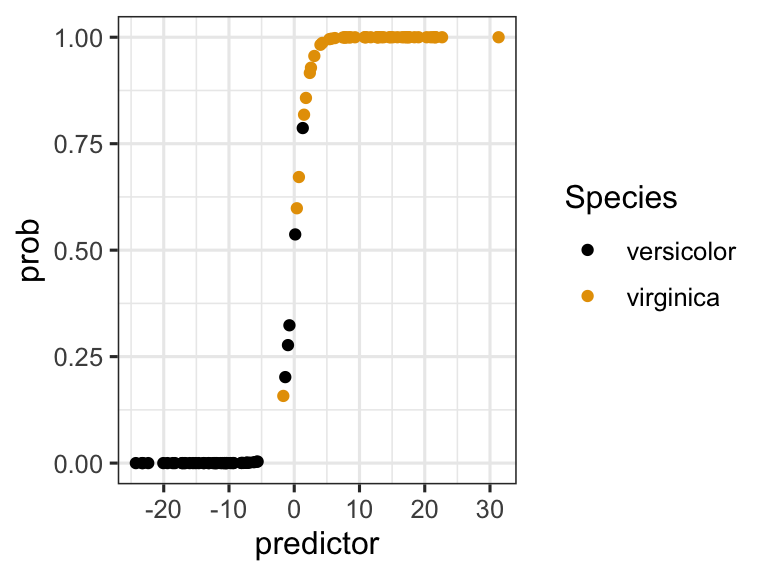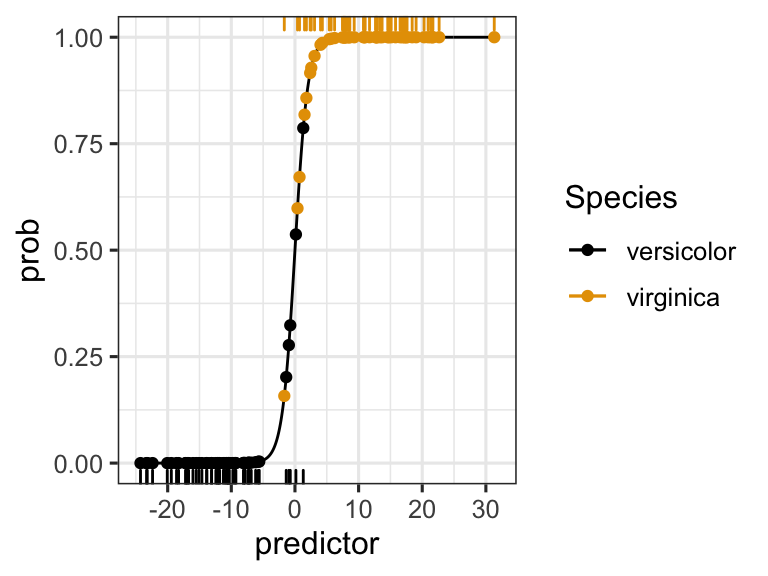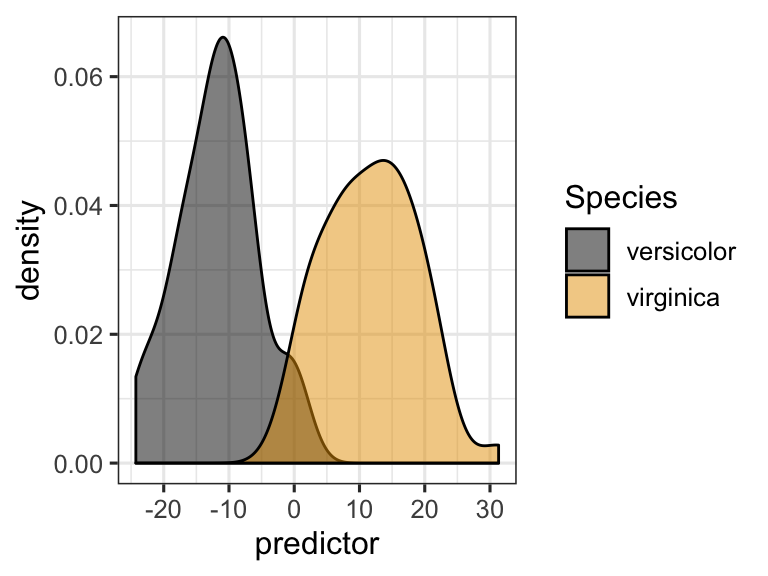## In-class worksheet 11

Feb 25, 2020

In this worksheet, we will use the library tidyverse and ggthemes:

library(tidyverse)
theme_set(theme_bw(base_size = 12)) # set default ggplot2 theme
library(ggthemes)

# 1. Fitting a logistic regression model to the iris data set

We will work with the iris data set. Specifically, with a subset of the data that consists only of the species virginica and versicolor:

# make a reduced iris data set that only contains virginica and versicolor species
iris_small <-
iris %>%
filter(Species %in% c("virginica", "versicolor"))

Fit a logistic regression model to the iris_small data set. Then successively remove predictors until only predictors with a p value less than 0.1 remain.

# logistic regression
glm_out <- glm(
Species ~ Sepal.Width + Sepal.Length + Petal.Width + Petal.Length,
data = iris_small,
family = binomial
) # family = binomial required for logistic regression

summary(glm_out)
##
## Call:
## glm(formula = Species ~ Sepal.Width + Sepal.Length + Petal.Width +
##     Petal.Length, family = binomial, data = iris_small)
##
## Deviance Residuals:
##      Min        1Q    Median        3Q       Max
## -2.01105  -0.00541  -0.00001   0.00677   1.78065
##
## Coefficients:
##              Estimate Std. Error z value Pr(>|z|)
## (Intercept)   -42.638     25.707  -1.659   0.0972 .
## Sepal.Width    -6.681      4.480  -1.491   0.1359
## Sepal.Length   -2.465      2.394  -1.030   0.3032
## Petal.Width    18.286      9.743   1.877   0.0605 .
## Petal.Length    9.429      4.737   1.991   0.0465 *
## ---
## Signif. codes:  0 '***' 0.001 '**' 0.01 '*' 0.05 '.' 0.1 ' ' 1
##
## (Dispersion parameter for binomial family taken to be 1)
##
##     Null deviance: 138.629  on 99  degrees of freedom
## Residual deviance:  11.899  on 95  degrees of freedom
## AIC: 21.899
##
## Number of Fisher Scoring iterations: 10

We remove Sepal.Length as it has the highest P value:

glm_out <- glm(
Species ~ Sepal.Width + Petal.Width + Petal.Length,
data = iris_small,
family = binomial
)
## Warning: glm.fit: fitted probabilities numerically 0 or 1 occurred
summary(glm_out)
##
## Call:
## glm(formula = Species ~ Sepal.Width + Petal.Width + Petal.Length,
##     family = binomial, data = iris_small)
##
## Deviance Residuals:
##      Min        1Q    Median        3Q       Max
## -1.75795  -0.00412   0.00000   0.00290   1.92193
##
## Coefficients:
##              Estimate Std. Error z value Pr(>|z|)
## (Intercept)   -50.527     23.995  -2.106   0.0352 *
## Sepal.Width    -8.376      4.761  -1.759   0.0785 .
## Petal.Width    21.430     10.707   2.001   0.0453 *
## Petal.Length    7.875      3.841   2.050   0.0403 *
## ---
## Signif. codes:  0 '***' 0.001 '**' 0.01 '*' 0.05 '.' 0.1 ' ' 1
##
## (Dispersion parameter for binomial family taken to be 1)
##
##     Null deviance: 138.629  on 99  degrees of freedom
## Residual deviance:  13.266  on 96  degrees of freedom
## AIC: 21.266
##
## Number of Fisher Scoring iterations: 10

Make a plot of the fitted probability as a function of the linear predictor, colored by species identity. Hint: you will have to make a new data frame combining data from the fitted model with data from the iris_small data frame.

lr_data <- data.frame(
predictor = glm_out$linear.predictors, prob = glm_out$fitted.values,
Species = iris_small$Species ) ggplot(lr_data, aes(x = predictor, y = prob, color = Species)) + geom_point() + scale_color_colorblind()If we want to plot the original observations as “rug” along the bottom or top of the plot, we first need to make two separate data frames with the data points for either species, and then feed them individually into the geom_rug() function. In addition, we make a data frame that contains the logistic function, so we get a smooth black line underneath the points. # extract virginica and versicolor data virg_data <- filter(lr_data, Species == "virginica") vers_data <- filter(lr_data, Species == "versicolor") # make data frame with logistic function predictor <- (-250:320)/10 prob <- exp(predictor)/(1 + exp(predictor)) log_fun_data <- data.frame(predictor, prob) # plot ggplot(lr_data, aes(x = predictor, y = prob, color = Species)) + geom_line(data = log_fun_data, color = "black") + geom_point() + geom_rug(data = vers_data, sides = 'b') + geom_rug(data = virg_data, sides = 't') + scale_color_colorblind()Make a density plot that shows how the two species are separated by the linear predictor. ggplot(lr_data, aes(x = predictor, fill = Species)) + geom_density(alpha = .5) + scale_fill_colorblind()# 2. Predicting the species Assume you have obtained samples from three plants, with measurements as listed below. Predict the likelihood that each of these plants belongs to the species virginica. plant1 <- data.frame( Sepal.Length = 6.4, Sepal.Width = 2.8, Petal.Length = 4.6, Petal.Width = 1.8 ) plant2 <- data.frame( Sepal.Length = 6.3, Sepal.Width = 2.5, Petal.Length = 4.1, Petal.Width = 1.7 ) plant3 <- data.frame( Sepal.Length = 6.7, Sepal.Width = 3.3, Petal.Length = 5.2, Petal.Width = 2.3 ) predict(glm_out, plant1, type = "response") ## 1 ## 0.6934611 Plant 1 has a 69% probability of being virginica. It may be a virginica. predict(glm_out, plant2, type = "response") ## 1 ## 0.06002675 Plant 2 has a 6% probability of being virginica. It is very likely versicolor. predict(glm_out, plant3, type = "response") ## 1 ## 0.9999943 Plant 3 has a 99.999% probability of being virginica. It is very likely virginica. # 3. If this was easy Pick a cutoff predictor value at which you would decide that a specimen belongs to virginica rather than versicolor. Calculate how many virginicas you call correctly and how many incorrectly given that choice. cutoff <- 0 virg_true <- sum(lr_data$predictor > cutoff & lr_data$Species == "virginica") virg_false <- sum(lr_data$predictor <= cutoff & lr_data$Species == "virginica") virg_true ##  49 virg_false ##  1 Now do the same calculation for versicolor. vers_true <- sum(lr_data$predictor <= cutoff & lr_data$Species == "versicolor") vers_false <- sum(lr_data$predictor > cutoff & lr_data\$Species == "versicolor")
vers_true
##  48
vers_false
##  2

If we define a call of virginica as a positive and a call of versicolor as a negative, what are the true positive rate (sensitivity, true positives divided by all possible positives) and the true negative rate (specificity, true negatives divided by all possible negatives) in your analysis?

tp <- virg_true/(virg_true + virg_false)
tn <- vers_true/(vers_true + vers_false)
tp
##  0.98
tn
##  0.96

The true-positive rate (sensitivity) is 98%.

The true-negative rate (specificity) is 96%.